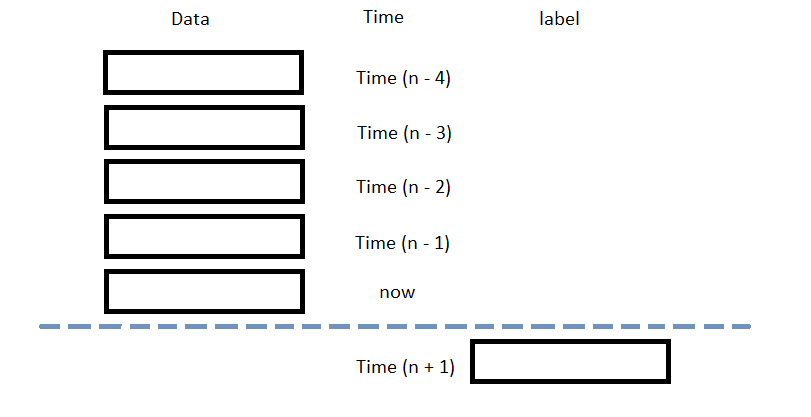# Popular Libraries

## Tensorflow

### Introduction

This page explains how to build, train, deploy and store Tensorflow v1 models. To view the tutorial on Tensorflow 2, see Keras.

### Import Libraries

Import the tensorflow libraries.

from AlgorithmImports import *
import tensorflow.compat.v1 as tf
import json5

tf.disable_v2_behavior()

You need the google.protobuf and json5 libraries to store and load models.

Disable tensorflow v2 behaviors in order to deploy a v1 model.

### Create Subscriptions

In the Initialize method, subscribe to some data so you can train the tensorflow model and make predictions.

self.symbol = self.AddEquity("SPY", Resolution.Daily).Symbol

### Build Models

In this example, build a neural-network regression prediction model that uses the following features and labels:

Data CategoryDescription
FeaturesThe last 5 closing prices
LabelsThe following day's closing price

The following image shows the time difference between the features and labels:Follow these steps to create a method to build the model:

1. Create a method to build the model for the algorithm class.
2. def BuildModel(self):
# Instantiate a tensorflow session
sess = tf.Session()

# Declare the number of factors and then create placeholders for the input and output layers.
num_factors = 5
X = tf.placeholder(dtype=tf.float32, shape=[None, num_factors], name='X')
Y = tf.placeholder(dtype=tf.float32, shape=[None])

# Set up the weights and bias initializers for each layer.
weight_initializer = tf.variance_scaling_initializer(mode="fan_avg", distribution="uniform", scale=1)
bias_initializer = tf.zeros_initializer()

# Create hidden layers that use the Relu activator.
num_neurons_1 = 32
num_neurons_2 = 16
num_neurons_3 = 8

W_hidden_1 = tf.Variable(weight_initializer([num_factors, num_neurons_1]))
bias_hidden_1 = tf.Variable(bias_initializer([num_neurons_1]))

W_hidden_2 = tf.Variable(weight_initializer([num_neurons_1, num_neurons_2]))
bias_hidden_2 = tf.Variable(bias_initializer([num_neurons_2]))

W_hidden_3 = tf.Variable(weight_initializer([num_neurons_2, num_neurons_3]))
bias_hidden_3 = tf.Variable(bias_initializer([num_neurons_3]))

# Create the output layer and give it a name, so it is accessible after saving and loading the model.
W_out = tf.Variable(weight_initializer([num_neurons_3, 1]))
bias_out = tf.Variable(bias_initializer())
output = tf.transpose(tf.add(tf.matmul(hidden_3, W_out), bias_out), name='outer')

# Set up the loss function and optimizers for gradient descent optimization and backpropagation.
# This example uses mean-square error as the loss function because the close price is a continuous data and uses Adam as the optimizer because of its adaptive step size.
loss = tf.reduce_mean(tf.squared_difference(output, Y))

return sess, X, Y, output, optimizer
3. Instantiate the model, input layers, output layer, and optimizer and then save them as class variables.
4. self.model, self.X, self.Y, self.output, self.optimizer = self.BuildModel(features, labels)
5. Call the run method with the result from the global_variables_initializer method.
6. self.model.run(tf.global_variables_initializer())

### Train Models

You can train the model at the beginning of your algorithm and you can periodically re-train it as the algorithm executes.

#### Warm Up Training Data

You need historical data to initially train the model at the start of your algorithm. To get the initial training data, in the Initialize method, make a history request.

training_length = 252*2
self.training_data = RollingWindow[float](training_length)
self.training_data.Add(trade_bar.Close)

#### Define a Training Method

To train the model, define a method that fits the model with the training data.

def get_features_and_labels(self, n_steps=5):
close_prices = list(self.training_data)[::-1]

features = []
labels = []
for i in range(len(close_prices)-n_steps):
features.append(close_prices[i:i+n_steps])
labels.append(close_prices[i+n_steps])
features = np.array(features)
labels = np.array(labels)

return features, labels

def my_training_method(self):
features, labels = self.get_features_and_labels()
self.model.run(self.optimizer, feed_dict={self.X: features, self.Y: labels})

#### Set Training Schedule

To train the model at the beginning of your algorithm, in the Initialize method, call the Train method.

self.Train(self.my_training_method)

To periodically re-train the model as your algorithm executes, in the Initialize method, call the Train method as a Scheduled Event.

# Train the model every Sunday at 8:00 AM
self.Train(self.DateRules.Every(DayOfWeek.Sunday), self.TimeRules.At(8, 0), self.my_training_method)

#### Update Training Data

To update the training data as the algorithm executes, in the OnData method, add the current close price to the RollingWindow that holds the training data.

def OnData(self, slice: Slice) -> None:
if self.symbol in slice.Bars:
self.training_data.Add(slice.Bars[self.symbol].Close)

### Predict Labels

To predict the labels of new data, in the OnData method, get the most recent set of features and then call the run method with new features.

new_features, __ = self.get_features_and_labels()
prediction = self.model.run(self.output, feed_dict={self.X: new_features[-1].reshape(1, -1)})
prediction = float(prediction.flatten()[-1])

You can use the label prediction to place orders.

if prediction > slice[self.symbol].Price:
self.SetHoldings(self.symbol, 1)
else:
self.SetHoldings(self.symbol, -1)

### Save Models

Follow these steps to save Tensorflow models into the Object Store:

1. Export the TensorFlow graph as a JSON object.
2. graph_definition = tf.compat.v1.train.export_meta_graph()
json_graph = json_format.MessageToJson(graph_definition)
3. Export the TensorFlow weights as a JSON object.
4. weights = self.model.run(tf.compat.v1.trainable_variables())
weights = [w.tolist() for w in weights]
json_weights = json5.dumps(weights)
5. Save the graph and weights to the Object Store.
6. self.ObjectStore.Save('graph', json_graph)
self.ObjectStore.Save('weights', json_weights)

You can load and trade with pre-trained tensorflow models that you saved in the Object Store. To load a tensorflow model from the Object Store, in the Initialize method, get the file path to the saved model and then recall the graph and weights of the model.

def Initialize(self) -> None:
if self.ObjectStore.ContainsKey('graph') and self.ObjectStore.ContainsKey('weights'):

# Restore the tensorflow graph from JSON objects
tf.reset_default_graph()
graph_definition = json_format.Parse(json_graph, tf.MetaGraphDef())
self.model = tf.Session()
tf.train.import_meta_graph(graph_definition)

# Select the input, output tensors and optimizer
self.X = tf.get_default_graph().get_tensor_by_name('X:0')
self.Y = tf.get_default_graph().get_tensor_by_name('Y:0')
self.output = tf.get_default_graph().get_tensor_by_name('outer:0')

# Restore the model weights from the JSON object.
weights = [np.asarray(x) for x in json5.loads(json_weights)]
assign_ops = []
feed_dict = {}
vs = tf.trainable_variables()
zipped_values = zip(vs, weights)
for var, value in zipped_values:
value = np.asarray(value)
assign_placeholder = tf.placeholder(var.dtype, shape=value.shape)
assign_op = var.assign(assign_placeholder)
assign_ops.append(assign_op)
feed_dict[assign_placeholder] = value
self.model.run(assign_ops, feed_dict=feed_dict)

The ContainsKey method returns a boolean that represents if the graph and weights is in the Object Store. If the Object Store doesn't contain the keys, save the model using them before you proceed.

### Clone Example Algorithm

You can also see our Videos. You can also get in touch with us via Discord.